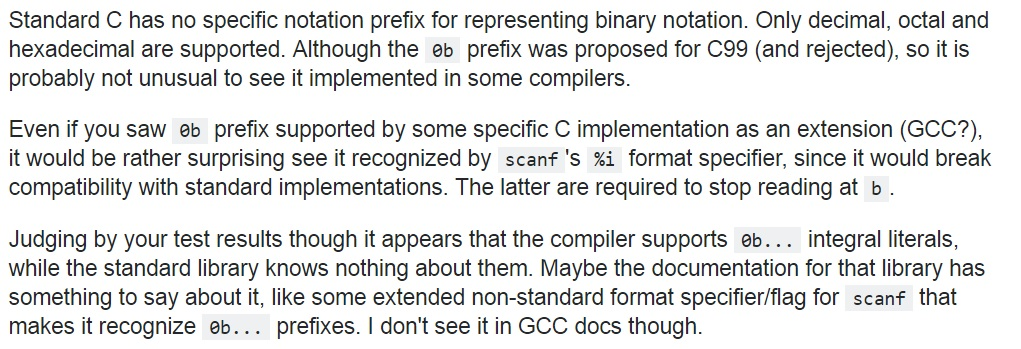ADVERTISEMENT

ADVERTISEMENT

ADVERTISEMENT

# How to assign binary value in a variable directly?

We have assigned Hexadecimal, Decimal, and Octal values in a variables in our many previous solutions.

Decimal value can be assigned directly (int a=100 ;), Octal value can be assigned by using "0" notation (int b=0144 ;) and Hexadecimal value can be assigned by using "0X" or "0x" notation (int c=0x64 ;).

First consider the following example, which is assigning Decimal, Octal and Hexadecimal values in variable and printing their values in Decimal format.

```#include <stdio.h>
int main()
{
int a=100;
int b=0144;
int c=0x64;

printf("a= %d\n",a);
printf("b= %d\n",b);
printf("c= %d\n",c);

return 0;
}
```

Output

```    a= 100
b= 100
c= 100
```

The values of a, b and c are 100 because, 0144 is the octal value of 100 and 0x64 is the Hexadecimal value of 100.

## How to assign Binary value in a variable?

Binary value can be assigned in a variable by using "0b" notation (we can say it format specifier too), this is a new feature which was introduced in C99 (not a standard feature, some compilers may not support this feature).

Let’s consider the following program

```#include <stdio.h>
int main()
{
int num=0b1010;
printf("Num: %d\n",num);
return 0;
}
```

Output

```    Num: 10
```

Remember: Read this post before using 0b notation to represent binary value
http://stackoverflow.com/questions/11597863/why-does-scanfi-a-not-take-binary-like-0b101ADVERTISEMENT

ADVERTISEMENT

Comments and Discussions

ADVERTISEMENT

ADVERTISEMENT

ADVERTISEMENT

ADVERTISEMENT

Languages: » C » C++ » C++ STL » Java » Data Structure » C#.Net » Android » Kotlin » SQL
Web Technologies: » PHP » Python » JavaScript » CSS » Ajax » Node.js » Web programming/HTML
Solved programs: » C » C++ » DS » Java » C#
Aptitude que. & ans.: » C » C++ » Java » DBMS
Interview que. & ans.: » C » Embedded C » Java » SEO » HR
CS Subjects: » CS Basics » O.S. » Networks » DBMS » Embedded Systems » Cloud Computing
» Machine learning » CS Organizations » Linux » DOS
More: » Articles » Puzzles » News/Updates

© https://www.includehelp.com some rights reserved.